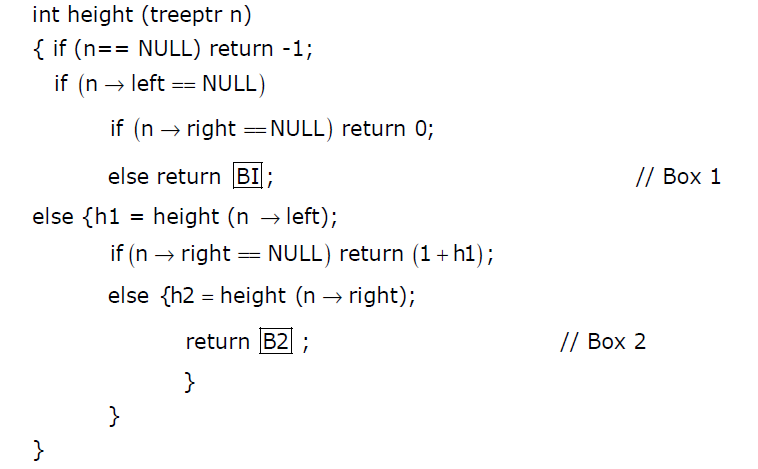Related Articles

# GATE | GATE CS 2012 | Question 45

• Difficulty Level : Hard
• Last Updated : 28 Jun, 2021

The height of a tree is defined as the number of edges on the longest path in the tree. The function shown in the pseudocode below is invoked as height (root) to compute the height of a binary tree rooted at the tree pointer root.The appropriate expression for the two boxes B1 and B2 are
(A) B1 : (1 + height(n->right)), B2 : (1 + max(h1,h2))
(B) B1 : (height(n->right)), B2 : (1 + max(h1,h2))
(C) B1 : height(n->right), B2 : max(h1,h2)
(D) B1 : (1 + height(n->right)), B2 : max(h1,h2)